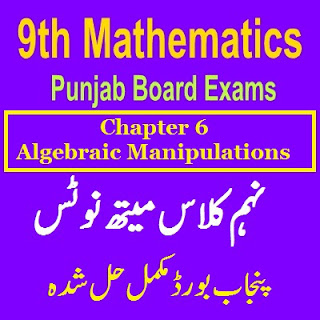# 9th Class Math Chapter 6 Algebraic Manipulation

Algebraic Manipulation Solved Exercises with Solutions of Chapter 6 Questions. If you have any help you can also comment us.

All solved notes of 9th class chapter wise and all Science Arts subjects solved Answers. Most important notes with solved MCQs Chapter wise also for the 9 Grade Students.Chapter 6 Mathematics Class 9th

Islamiat, Mathematics, English, Urdu, Pakistan Studies, General Mathematics Notes are also Available for 9 class students and teachers help guides.

9th Mathematics Notes Algebraic Manipulation

In this Unit 6 Algebraic Manipulation you will learn about Highest Common Factors H.C.F and Least Common Multiple LCM of Algebraic Expressions Highest Common Factor. HCF.

All Definitions are also explained in this section of algebraic manipulation.

If two or more then algebraic expressions are given then their common factor of highest power is call HCF of the expressions.

Least Common Multiple LCM

If an algebraic expression p(x) is exactly divisible by two or more expressions, then p(x) is caled the common multiple of the given expressions.

The Least commo Multiple LCM is the product of the common factor of the given expression.

Square Root Definition: Square Root of an algebraic expression px will be another algebraic expression qx if [q(x)] X [q(x)] = p(x)

Online MCQs Quiz Tests all subjects for Online tests preparations with topic wise Learning.

Previous Post
Next Post